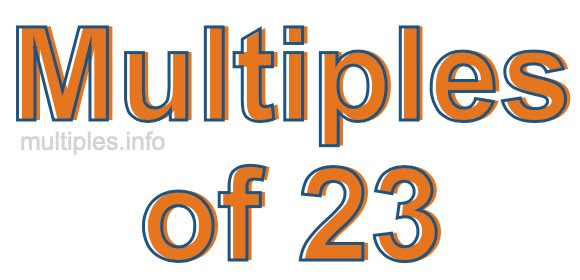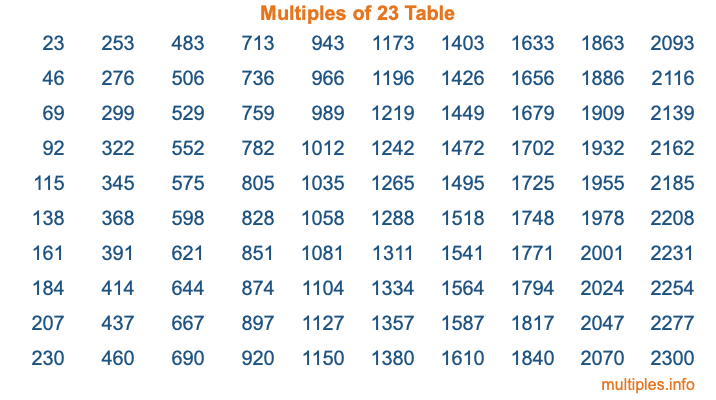Multiples of 23Welcome to the Multiples of 23 page. Here we will first teach you everything you will ever need to know about the multiples of 23, and then give you a study guide summary of everything we taught you to make sure you remember it all. Use this page to look up facts and learn information about the multiples of 23. This page will make you a multiples of twenty-three expert!

Definition of Multiples of 23
Multiples of 23 are all the numbers that when divided by 23 equal an integer. Each of the multiples of 23 are called a multiple. A multiple of 23 is created by multiplying 23 by an integer.

Therefore, to create a list of multiples of 23, you start with 1 multiplied by 23, then 2 multiplied by 23, then 3 multiplied by 23, and so on for as long as you want. Thus, the list of the first five multiples of 23 is 23, 46, 69, 92, and 115. To see a larger list of multiples of 23, see the printable image of Multiples of 23 further down on this page. We also have a category where you can choose any nth multiple of 23.

Multiples of 23 Checker
The Multiples of 23 Checker below checks to see if any number of your choice is a multiple of 23. In other words, it checks to see if there is any number (integer) that when multiplied by 23 will equal your number. To do that, we divide your number by 23. If the the quotient is an integer, then your number is a multiple of 23.

Is  a multiple of 23?

Least Common Multiple of 23 and ...
A Least Common Multiple (LCM) is the lowest multiple that two or more numbers have in common. This is also called the smallest common multiple or lowest common multiple and is useful to know when you are adding our subtracting fractions. Enter one or more numbers below (23 is already entered) to find the LCM.

Check out our LCM Calculator if you need more details about the Least Common Multiple or if you need the LCM for different numbers for adding and subtraction fractions.

nth Multiple of 23
As we stated above, 23 is the first multiple of 23, 46 is the second multiple of 23, 69 is the third multiple of 23, and so on. Enter a number below to find the nth multiple of 23.

th multiple of 23

Multiples of 23 vs Factors of 23
23 is a multiple of 23 and a factor of 23, but that is where the similarities end. All postive multiples of 23 are 23 or greater than 23. All positive factors of 23 are 23 or less than 23.

Below is the beginning list of multiples of 23 and the factors of 23 so you can compare:

Multiples of 23: 23, 46, 69, 92, 115, etc.

Factors of 23: 1, 23

As you can see, the multiples of 23 are all the numbers that you can divide by 23 to get a whole number. The factors of 23, on the other hand, are all the whole numbers that you can multiply by another whole number to get 23.

It's also interesting to note that if a number (x) is a factor of 23, then 23 will also be a multiple of that number (x).

Multiples of 23 vs Divisors of 23
The divisors of 23 are all the integers that 23 can be divided by evenly. Below is a list of the divisors of 23.

Divisors of 23: 1, 23

The interesting thing to note here is that if you take any multiple of 23 and divide it by a divisor of 23, you will see that the quotient is an integer.

Multiples of 23 Table
Below is an image of the first 100 multiples of 23 in a table. The table is in chronological order, column by column. The first column has the first ten multiples of 23, the second column has the next ten multiples of 23, and so on.The Multiples of 23 Table is also referred to as the 23 Times Table or Times Table of 23. You are welcome to print out our table for your studies.

Negative Multiples of 23
Although not often discussed or needed in math, it is worth mentioning that you can make a list of negative multiples of 23 by multiplying 23 by -1, then by -2, then by -3, and so on, to get the following list of negative multiples of 23:

-23, -46, -69, -92, -115, etc.

Multiples of 23 Summary
Below is a summary of important Multiples of 23 facts that we have discussed on this page. To retain the knowledge on this page, we recommend that you read through the summary and explain to yourself or a study partner why they hold true.

There are an infinite number of multiples of 23.

A multiple of 23 divided by 23 will equal a whole number.

23 divided by a factor of 23 equals a divisor of 23.

The nth multiple of 23 is n times 23.

The largest factor of 23 is equal to the first positive multiple of 23.

23 is a multiple of every factor of 23.

23 is a multiple of 23.

A multiple of 23 divided by a divisor of 23 equals an integer.

23 divided by a divisor of 23 equals a factor of 23.

Any integer times 23 will equal a multiple of 23.

Multiples of a Number
Here you can get the multiples of another number, all with the same attention to detail as we did for multiples of 23 on this page.

Multiples of
Multiples of 24
Did you find our page about multiples of twenty-three educational? Do you want more knowledge? Check out the multiples of the next number on our list!